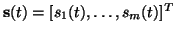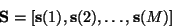Next: EM-algorithm for Independent Component Up: Fast Algorithms for Bayesian Previous: Fast Algorithms for Bayesian

# Introduction

We consider the problem of finding linearly mixed source signalsfrom observed noisy linear mixtures

x(t)=As(t)+n(t).

The mixing matrix A is unknown and n(t) is additive noise. When we consider a finite number of observed mixture samples, we may write the data model in matrix form as

X=AS+N

Each time-indexed matrix contains a sequence of vector samples, e.g.To find the mixing matrix A, it is necessary that the source signals possess certain statistical properties. For example, it is sufficient that the source signals are not Gaussian. It is also sufficient that the possibly Gaussian sources have time dependencies, together with some other conditions.

Harri Lappalainen
2000-03-09